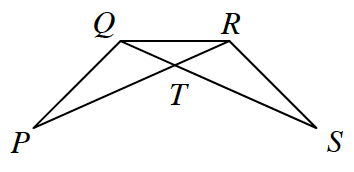### Home > CCG > Chapter 8 > Lesson 8.1.3 > Problem8-30

8-30.In the figure at right, if $PQ = SR$ and $PR = SQ$, prove that $\angle P \cong \angle S$. Write your proof either in a flowchart or in two-column proof form.

Statement

Reason

$PR=SQ$

Given

$PQ=RS$

Given

$QR=QR$

Reflexive

$ΔPQR≅ΔSRQ$

$\text{SSS}≅$

$∠P≅∠S$

$≅Δ\text{s}→≅\text{Parts}$

Be sure to look at the reasoning and understand why this proof is organized as it is.